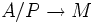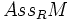# Associated prime to a module

Let$R$ be a commutative unital ring and$M$ be a$R$-module. A prime ideal$P$ of$R$ is said to be associated to$M$ if it satisfies the following equivalent conditions:
•$P$ is the annihilator of an element of$M$
• There is an injective homomorphism$A/P \to M$ of$A$-modules
The set of all primes associated to$M$ is denoted as$Ass_RM$.